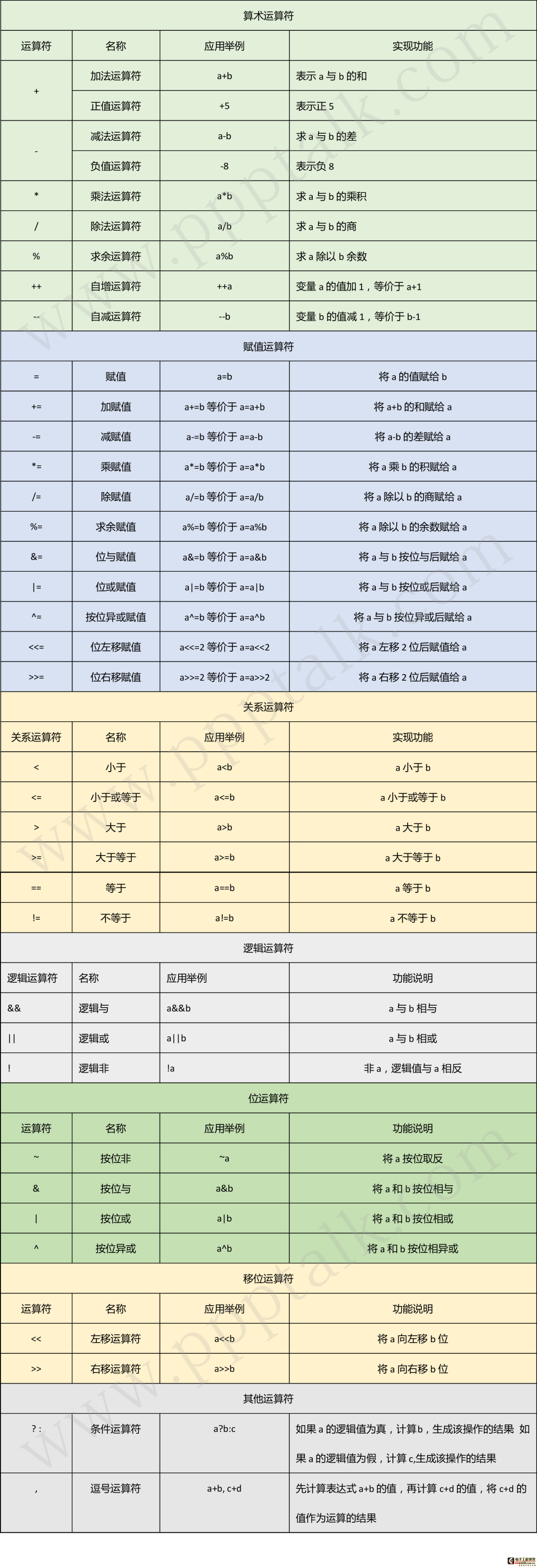# 06-运算符大全-c语言-值得收藏

## 算术运算符

+（加）   -（减）  *（乘）  /（除）   %（取余/取模）


++（自增）   --（自减）


### 自增与自减

int i = 4;

i--;
printf("i = %d", i);
// 输出 i = 3

i++;
printf("i = %d", i);
// 输出 i = 4



i++;的含义是i = i + 1;

i--;的含义是i = i - 1;

### 自增自减中运算与赋值的先后顺序

int i = 4;
int j = i--;
// --在后，所以先将i赋值给j，然后i再-1
printf("i = %d, j = %d", i, j); // 输出 i = 3, j = 4

int a = 4;
int b = --i;
// --在前，所以i先-1，然后再将i赋值给j
printf("a = %d, b = %d", a, b); // 输出 a = 3, b = 3



## 三目运算符

表达式1 ? 表达式2 : 表达式3


int a = 1;
int b = a > 5 ? 10 : 20;
printf("b = %d", b); // 输出20



用三目运算符求三个数的最大值。


## 关系运算符

>（大于）  <（小于）
>=（大于等于）  <=（小于等于）
==（等于）  !=（不等于）


## 逻辑运算符

&&（与）  ||（或）  !（非）


int a = 1;
printf("!a = %d\n", !a); // 输出0

int b = 3, c = 10;
printf("b && c = %d\n", b && c); // 输出1

int d = 3, e = 0;
printf("d && e = %d\n", d && e); // 输出0

int f = 3, g = 10;
printf("f || g = %d\n", f || g); // 输出1

int h = 3, i = 0;
printf("h || i = %d\n", h || i); // 输出1



•!（非）：!真 = 假!假 = 真•&&（与）：有0则0•||（或）：有1则1

•在与运算中，如果前面的表达式为假，则不执行后面的表达式•在或运算中，如果前面的表达式为真，则不执行后面的表达式

int a = 10, b = 20;
a < 10 && b++;
printf("a = %d, b = %d", a, b); // 输出a = 10, b = 20

// 同理
a < 10 || b++;
printf("a = %d, b = %d", a, b); // 输出a = 10, b = 21



## 位运算符

### 位逻辑运算符

|（按位或）  &（按位与）  ~（按位取反）  ^（按位异或）


int a = 5 | 3;
/* 表示为：
101
011
---------
111
*/
printf("a = %d", a); // 输出 a = 7



### 移位运算符

>>（右移）  <<（左移）


int a = 3 << 2;
/*

3的二进制表示为：011
3 << 2的含义是：将3向左移2位，表示为：01100

*/
printf("a = %d", a); // 输出a = 12



<<（左移）：放大2^n倍
（右移）：缩小2^n倍


## 逗号运算符

C语言中，多个表达式可以用逗号分开，其中用逗号分开的表达式的值分别结算，但整个表达式的值是最后一个表达式的值。逗号运算符优先级最低。

int a = 10, b = 20;
int x, y;
x = (a++, b);
y = (x = x + 1, 10);
printf("x = %d, y = %d, a = %d, b = %d", x, y, a, b);
// 输出x = 21, y = 10, a = 11, b = 20《c语言简明教程》

01-C语言的过去与未来

02-Dev C++  安装及使用教程

03-C语言的第一个简单实例

04-关键字与标识符-C语言

05-数据类型与常量变量-C语言IT入门 感谢关注 程序员题库→ 程序员用的单词表→ 练习地址：www.520mg.com/it

↓↓点击下方阅读原文，下载高清总结图

08-01129
03-1825506-141万+
10-299
11-301
07-041万+
05-11189
07-11606
05-05115